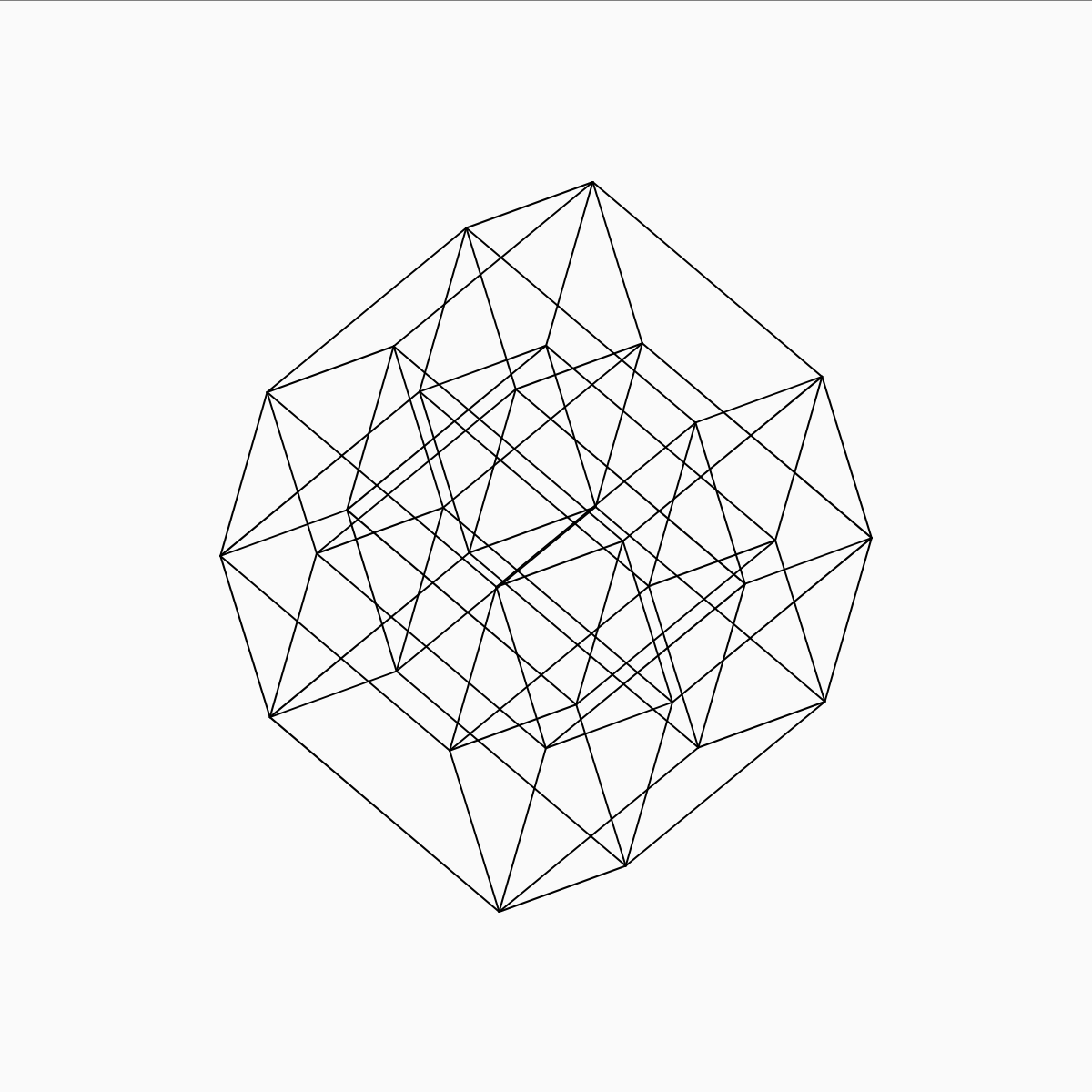### Hypercube Visualization

This project was a Java-based simulation allowing one to visualize wireframe hypercubes—i.e., the equivalent of cubes in more than three dimensions. The simulation asks for the user to input the number of dimensions, n. This could be any positive integer. It then uses the mathematical definition of an n-cube to generate its vertices and edges in n-dimensional space. To allow us to see the hypercube from any angle, a generalized n-dimensional rotation is performed. Finally, the geometry is projected axonometrically into two dimensions before it is displayed to the screen.

This project was a chance for me to learn Java, and dates to before I did a lot of prototyping in nicer environments like Processing. Seen here is a Python port of the code, showing a 5-dimensional hypercube.